Select Page

# Understanding optical invariants

by | Apr 30, 2019 | optics definitions

Optical invariants are mathematical expressions representing several optical parameters. The values for each optical invariant are constant despite the fact that optical parameters change from one part or element of the optical system to another.

Optical invariants are significant during optical systems design because they make it possible to achieve quicker results while avoiding mistakes in optical paramters’ calculations.

There are 4 main invariants of optical systems:

1. Lagrange-Helmholtz invariant for paraxial optics

2. Abbe sine condition for high aperture systems

3. Straubel invariant for illuminating optical systems with light pencils transformation

4. Etendue invariant for illuminating optical systems with extended source

## Lagrange-Helmholtz invariantA schematic optical scheme is shown with the help of principal planes

Fig 1 presents an optical schematic shown with the help of principal planes ℎ and ℎ′.
Symbols on the scheme:

• 𝑦 and 𝑦′ are heights of object and image
• 𝛼 and 𝛼’ aperture angles in object and image spaces
• 𝑛 and 𝑛’ are indices of refraction in object and image spaces

In case of small angles and ray heights, the relation between these parameters is represented below.This is a well known invariant of Lagrange-Helmholtz. It shows that the product of aperture angle and field height is constant in image and object space. This relation works for any optical system with any number of optical components.

This invariant defines the relation between image aperture angle and image height when the object aperture angle and height are known and fixed. The main optical parameters of the optical system can then be defined.

In an existing optical system, Lagrange-Helmholtz makes it possible to estimate the relation between two pair parameters of object and image.

### Modifications of Lagrange-Helmholtz invariant for different methods of object to image conjugation

#### Infinite to finite conjugation

Example: Objective lens of cameraInfinite to finite conjugation-objecitve camera lens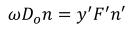Where ω is field angle, D0/2 is half of aperture diameter, F is f-number.

#### Infinite to infinite conjugation

Example: Galilean telescope systemInfinite to finite conjugation-Galilean telescopeWhere ω is field angle, D0/2 is half of aperture diameter, F is f-number.

### Lagrange-Helmholtz invariant application

Lagrange-Helmholtz invariant is very important for estimating the relation of parameters of systems in paraxial region, where apertures and fields of view are quite small.

This invariant defines the main parameters of paraxial optical systems: focal lengths, magnification, positions of object and image, entrance and exit pupil, and principal planes.

## Abbe sine conditionAbbe sine condition

Lagrange-Helmholtz invariant is true for any optical system with relatively small y (ω) and α. For an optical system where essential α is similar to a microscope lens, a more accurate invariant is needed, the Abbe sine condition.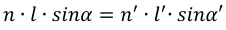Comparison of Lagrange-Helmholtz invariant with Abbe sine condition shows that Sine condition uses the sinus of aperture angles instead of their values.

### Abbe sine condition application

Sine condition is true for optical systems that don’t have spherical aberrations and coma. Vice versa, if sine condition is true for a given optical system, that system doesn’t have spherical aberration and coma. It is named aplanatic. In other words, sine condition is a condition of aplanatism. This is a key indicator of a microscope lens performance.

If the optical system has residual aberrations, this can be characterized as OSC (offense against the sine condition). The level of deviation from sine condition shows the extent of proximity of the concerned optical system to an aplanatic one.

One effect from sine condition is that the maximal aperture aplanatic systems is 0.5, though non achievable.

## Light pencil invariant and Straubel theorem for transferringLight pencil invariant and Straubel theorem for transferring

Let’s consider the propagation of a light pencil beam through the refractive surface (Fig. 5). The volume of a light pencil is limited by surfaces 𝑑𝑆1, 𝑑𝑆 and the marginal rays between them. Two light pencil beams are presented on Fig. 5:

1. Between 𝑑𝑆1 surface and refractive surface 𝑑𝑆

2. Between refractive surface 𝑑𝑆 and surface 𝑑𝑆2

Rays propagating between surfaces form several solid angles are 𝑑Ω, 𝑑Ω1, and 𝑑Ω2. The light pencil invariant demonstrates the interrelation of these angles and surfaces: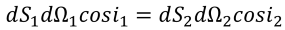Straubel theorem takes into account the media refraction coefficient with different indices refraction 𝑛 and 𝑛’.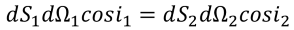This equation is valid for any number of reflections and/or refractions.

## Etendue invariantEtendue invariant

A real optical system works with extended sources with square 𝑆 and finite apertures determined by the sizes and system construction. The light spot area generated by rays passed through the optical system is determined by the Etendue invariant: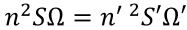where S and S’ are areas of source and its image and Ω is the solid angle which is defined as follows: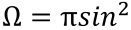where 𝑢 is aperture angle.

Accordingly, the Etendue invariant is: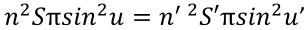### Etendue invariant application

#### The limit of light spot size

We can use the Etendue invariant to find the ratio of source area and the light spot area formed with the help of optics.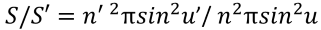The maximum value of sine function for the image aperture angle is 1 when the angle 𝑢’=90°. In this case, the ratio will have a maximum value (𝐶𝑚𝑎𝑥). Its formula is: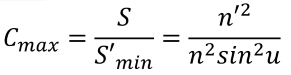#### Throughput of LED illumination optical system

If the optics are not large enough to overcome the source Etendue, there will be an additional efficiency loss. In this case,the source Etendue will be limited by optics Etendue; the Etendue invariant will not work. It can however help in finding loss levels and even in optimizing them with the help of this formula: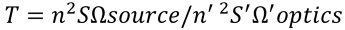Need assistance designing a custom optic or imaging lens ? Learn more about our design services here.